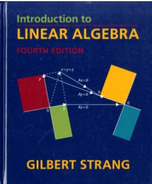Introduction To Linear Algebra - 4 Edition - Chapter 3.2 - Problem 37
Register Now

Join StudySoup

Get Full Access to Introduction To Linear Algebra - 4 Edition - Chapter 3.2 - Problem 379780980232714

# Kirchhoff's Law says that current in = current out at every node. This network has six

Introduction to Linear Algebra | 4th Edition

Problem 37

Kirchhoff's Law says that current in = current out at every node. This network has six currents Yl, ... ,Y6 (the arrows show the positive direction, each Yi could be positive or negative). Find the four equations Ay = 0 for Kirchhoff's Law at the four nodes. Find three special solutions in the nullspace of A.

Accepted Solution
Step-by-Step Solution:
Step 1 of 3

Parallelogramole a 7 I j i Components LetP Cxy and Q xz.gr Draw vectorJ EQ y ie l J Xa Xi y ga g yz p y y The components of v cerev Xa Xi and v ya ly Then I v cuz yr 9 J v 27 µ x Magnitudeof J Gl Five I'lllengthmagnitudefvector u µ 4 U2 1412 uz Ui U s Any vector 8 oflength1 Gil c is l ful lil JI

###### Chapter 3.2, Problem 37 is Solved

Step 2 of 3

Step 3 of 3

Unlock Textbook Solution